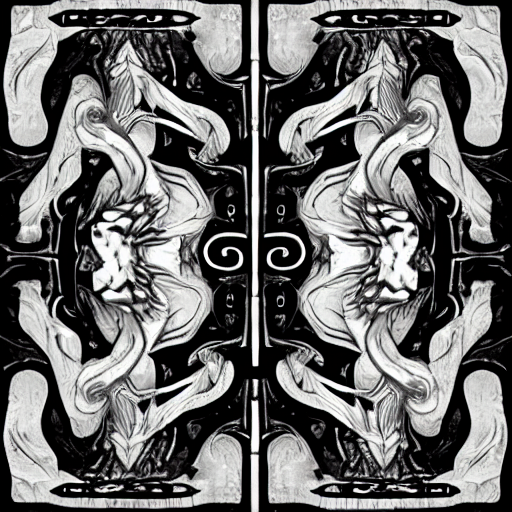### Duality done right

A more elegant view of duality then is presented in axler's book

Update: Axler mentions some of these results in exercises 34-37. But I feel he doesn't given them as much emphasis as they deserve.

Update 2: Re-reading my hot take after doing some functional analysis, I still somewhat agree but the tone I used was too strong. Talking about dimension can convey when results don't hold in general, though many of the lemmas in Axler can easily be extended to infinite-dimension the main theorems can't.

Update 3: With more perspective, this post is a result of me trying to find a natural isomorphism between the dual & primal vector spaces, and coming to a better appreciation of exactly where the trouble is.

## Notation

Let $V,W$ be finite-dimensional vector spaces. $U$ denotes a subspace of $V$, $T \in \mathcal L(V,W)$ denotes a linear transformation from $V$ to $W$, $R(T)$ and $N(T)$ denote the range and nullspace of $T$ respectively.

## Fundamental Theorem

Axler presents the fundamental theorem of linear maps as

$\dim V = \dim R(T) + \dim N(T) \tag{1}$

However, a more profitable way to view it is via the isomorphism

$\tilde T(v + N(T)) = Tv$

It's easy to see $\tilde T$ is injective and surjective, hence $\tilde T$ is bijective, meaning $V/N(T) \cong R(T)$

This implies (1) after taking the dimension of both sides and turning the quotient into a subtraction. However the isomorphism approach has several advantages

1. It's more intuitive to think about isomorphisms then dimensions being equal. dimension doesn't convey structure, it's bad for the same reason determinants are bad.
2. It shows that the fundamental theorem of linear maps is a special case of the first isomorphism theorem.
3. It generalizes to infinite dimensional vector spaces (the first isomorphism theorem doesn't care about dimension and neither should you)

## Duality

NOTE: This should be mostly review for my target audience

Let $V$ be a vector space, define the dual space $V' = \mathcal L(V, \mathbf F)$ and the dual map $T'(\varphi) = \varphi \circ T$. if $U \subseteq V$ define the annihilator as

$U^0 = \{\varphi \in V' : \varphi(u) = 0 \text{ for all u \in U}\}$

The annihilator is a bridge between the primal and the dual because of two key facts

1. (a) $N(T') = (R(T))^0$ and (b) $R(T') = (N(T))^0$
2. $V'/U^0 \cong U'$

Remark: (1b) starts the bridge from $R(T')$ to $R(T)$ and (2) finishes it by allowing us to remove the annihilator via an isomorphism to $R(T)'$ (we'll see more about this later).

Proof: most of (1) is clear after thinking about the definitions, to see $(N(T))^0 \subseteq R(T')$ see Axler 3.109 (the proof uses dimension, and I think only holds on finite dimensional vector spaces, sad.)

For (2) let $i \in \mathcal L(U, V)$ be the defined by $i(u) = u$ and apply the fundamental theorem on the dual $i' : V' \to U'$ to get

$V'/N(i') \cong R(i')$

We see $N(i') = U^0$ from (1a) and $R(i') = U'$ from (1b) giving the desired result.

These two facts allow us to construct a bridge of isomorphisms between the primal and the dual

$R(T') \cong W'/N(T') = W'/(R(T))^0 \cong R(T)' \cong R(T)$

The first holds by the fundamental theorem, the second holds by (2), the third holds by (3) and the last holds by the isomorphism with the dual basis.

Using these equivalences we can prove several things very easily, for example these two theorems

1. $T$ injective iff $T'$ surjective
2. $T$ surjective iff $T'$ injective

Are easily seen by considering isomorphisms from the chain above

1. $V/N(T) \cong R(T')$
2. $R(T) \cong V'/N(T')$

Plugging in the cases easily gives the desired results

• If $N(T) = \{0\}$ then $V/N(T) = V \cong R(T')$ implies $R(T') = V'$
• If $R(T') = V'$ then $V' \cong V/N(T)$ implies $N(T) = \{0\}$
• If $R(T) = V$ then $V \cong V'/N(T')$ implies $N(T') = \{0\}$
• If $N(T') = \{0\}$ then $V' \cong R(T)$ implies $R(T) = V$

TODO: Should only need two cases since $T'' = T$

I'm skipping details, but the core ideas are all here, you can reconstruct the full proofs with no extra leaps of insight needed.